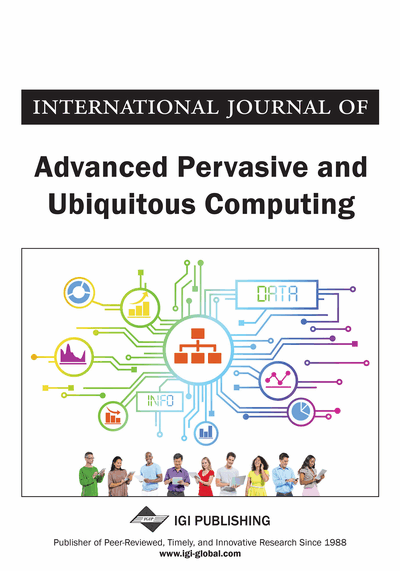# Time Series Forecasting as a Measure

Liu Hongcong (Henan Science and Technology Career Technical College, Henan, China)
DOI: 10.4018/japuc.2013040105
Available
\$37.50
No Current Special Offers

## Abstract

In this paper, the time series prediction is as a measure. At the same time, the optimal combination forecast using each method can be defined as the actual impact measurement value of true. Effect of its theoretical estimation has error correlation coefficient values. The optimal weighted linear combination is the theoretical prediction which can be proved, also, simple averaging method is linear combination forecasting optimal weights. Especially, based on the robust statistic theory, the mathematical derivation and numerical tests on the superiority is simple.
Article Preview
Top

## The Combined Forecasting

Actual complex time series forecasting is a difficult problem. Complex combined forecasting method, which is proposed by J. M. Bates and C. W. J. Granger in 1969, is the actual time series forecasting a new stage of development. The linear combined forecasting can be expressed as shown below.

For the time series, t = 1, 2, …,N methods using the predicted results:, i=1, 2,… N, t=1,2, …, T (1)

In general, we do not assume thatis normally distributed.obey arbitrary distribution. It because that we are difficult to determine the specific distribution pattern of the noise an forecasting error. Thought assumed to follow a normal distribution is reasonable, when the T is larger.

The results of the linear combined forecasting is:, t=1,2, …, T (2)is the residual of a forecasting result. Its mean may not 0, when the linear combined forecasting is biased. In this case, we can analyze the historical data to estimate the residual mean of, and then to be corrected. The variance of the residualgenerally consists of two parts. One part is the noise in the historical data, which can’t been forecasted. Another part comes from the forecasting of the defect. Any kind of forecasting method has its own shortcomings, a single method to predict will cause additional errors. The combined forecasting is powerless for the first part factor, and it forms predicted limits. We can improve the forecasting methods and use of combined forecasting, which can significantly reduce the forecasting error.

Top

## Impact Factors Of The Combined Forecasting

The main factors affecting combined forecasting accuracy rate is:

## Complete Article List

Search this Journal:
Reset
Open Access Articles: Forthcoming
Volume 11: 4 Issues (2019)
Volume 10: 4 Issues (2018)
Volume 9: 4 Issues (2017)
Volume 8: 4 Issues (2016)
Volume 7: 4 Issues (2015)
Volume 6: 4 Issues (2014)
Volume 5: 4 Issues (2013)
Volume 4: 4 Issues (2012)
Volume 3: 4 Issues (2011)
Volume 2: 4 Issues (2010)
Volume 1: 4 Issues (2009)
View Complete Journal Contents Listing